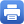Columbia Home
Fall 2019 Minerva Lectures

Come join us on Friday, September 27th, October 4th and 11th from 3:15 – 5pm in room 407. Starting Friday, September 27, 2019 Professor Makiko Sasada (University of  Tokyo), will be giving a special lecture titled;

### The Box-ball System, Discrete Integrable Systems and Generalized Pitman’s Transform

The Korteweg-de Vries equation (KdV equation) and the Toda lattice are fundamental examples of classical integrable systems. These integrable systems with random initial measures have been intensively studied in recent years.

In this series of lectures, I will present our recent results on discrete versions of the KdV equation and the Toda lattice starting from random initial conditions. As a fundamental example, in the first lecture, I will focus on the box-ball system (BBS). The model, introduced by Takahashi and Satsuma in 1990, is a cellular automaton that exhibits solitonic behaviour, and can be understood as a special case of the ultra-discrete KdV equation and the ultra-discrete Toda lattice. The BBS is also known to be obtained from a vertex model by crystallization. I will show a new description of the BBS dynamics using Pitman’s transform of a simple random walk path, and give several results related to the BBS with random initial measures.

In the second and third lectures, I will introduce some generalizations of Pitman’s transform and show that the dynamics of several discrete integrable systems, such as the discrete KdV equation, the ultra-discrete KdV equation, the discrete Toda lattice and the ultra-discrete Toda lattice are given by them. This observation is applied to define the dynamics uniquely on the infinite configuration space and study the invariant measures. Some analogy between the discrete Toda lattice (resp. ultra-discrete Toda lattice) and the random polymers (resp. the last passage percolation) and the role of Burke’s theorem will be also discussed.

The lectures are based on the joint work with David Croydon, Tsuyoshi Kato and Satoshi Tsujimoto.”

Friday, September 27th, October 4th and October 11th from 3:15pm – 5pm

Mathematics Hall, room 407

Tea will be served at 3:00 p.m.

*Minerva Lecture Flyer*Print this page